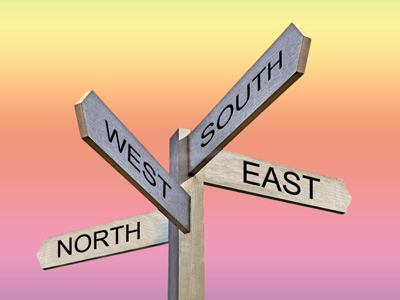North, East, South and West are found at 0o, 90o, 180o and 270o.

# Position 2 (Difficult)

This is the second quiz in our Difficult section on Position. It contains a mix of things you will have learned when you played our previous Eleven plus maths quizzes on the topic.

To get all the questions right you will need to understand the number of degrees in a complete rotation, a half rotation, a quarter rotation and an eighth of a rotation. You’ll also need to know all about where coordinates are placed on a graph and the difference between x and y axis, as well as the four quadrants.

Sounds like a lot doesn’t it? But, if you have played our easy and difficult quizzes, I’m sure you’ll breeze through it.

1.
Which direction does the straight line starting at (1, 1) and ending at (-1, 3) point in?
NE
N
NW
W
A diagram will help a lot in doing these questions!
2.
How many half rotations are there in 1,260o?
7
9
12
14
There are 1,260o ÷ 180o = 7 half rotations
3.
What are the coordinates of the point that is the same distance away from the y-axis as the point (4, 0)?
(-4, 0)
(0, 4)
(0, 0)
(2, 4)
(4, 0) is at 4 on the positive x-axis: 4 units to the right the y-axis. (-4, 0) is at -4 on the negative x-axis: 4 to the left of the y-axis
4.
Which coordinates are SE of (1, 3)?
(-1, 5)
(1, -5)
(5, -1)
(-5, 1)
Starting at (1, 3) draw a line 135o from North. All points along this line (including 5, -1) lie SE of (1, 3)
5.
You are facing SW. If you turn through 90o anticlockwise and then 135o clockwise, which direction will you be facing now?
NW
W
SW
S
'Anticlockwise' means that you turn (rotate) in the opposite direction to which the hands of the clock turn: the hands of the clock turn 'clockwise'. 90o anticlockwise makes you face SE. Then, 135o = three lots of 45o clockwise, which makes you face W
6.
A circle with centre (3, -3) and diameter 8 cm is drawn on the coordinate plane. Which points will the circle pass through?
(3, -1), (7, 3), (3, 7), (-1, -3)
(3, 1), (7, -3), (3, -7), (-1, -3)
(-3, 1), (-7, 3), (3, -7), (-1, -3)
(3, 1), (7, -3), (-3, 7), (1, -3)
The radius is half the diameter = 4 cm; therefore, the circle will pass through those points that are at a distance of 4 cm from its centre (3, -3)
7.
If you draw out a straight line path starting at A (1, 3) and move as follows: 5 cm E to point B, then SE to point C, which is due South of point A. From there you move 6 cm N to point E, where you stop. What are the coordinates of point E?
E = (1, 4)
E = (4, 4)
E = (4, 1)
E = (1, 1)
The best way to work this out is with a diagram – it’s very hard to keep all that information in your head!
8.
Which of the following is the angle between SW and N?
360o
135o
225o
315o
Seven lots of 45o which is the same as NW
9.
You are facing E. If you turn through 315o anticlockwise and then 225o clockwise, which direction will you be facing now?
W
NW
N
NE
315o anticlockwise = seven lots of 45o (seven-eighths of a turn) makes you face SE. 225o clockwise = 5 lots of 45o = (five-eighths of a turn) makes you face N
10.
If you join up the points A (-2, 0), B (-2, 2), C (0, 2), D (0, 0) with straight lines you will get a square that lies in a certain quadrant. If the square is rotated anticlockwise through 90o about the origin (0, 0), which quadrant will it end up in and what will its new coordinates be?
A (0, 2), B (2, 2), C (2, 0), D (0, 0) in the first quadrant
A (0, 2), B (-2, 2), C (-2, 0), D (0, 0) in the third quadrant
A (0, -2), B (2, -2), C (2, 0), D (0, 0) in the fourth quadrant
A (0, -2), B (-2, -2), C (-2, 0), D (0, 0) in the third quadrant
The square is initially in the second quadrant. Join up the four corners with straight lines (the lengths of which should be 2 units) to the centre of rotation (0, 0). Now rotate the lines and watch where their end points end up: these will give you the positions of the new coordinates of the square. Draw it!
Author:  Frank Evans